\$9.00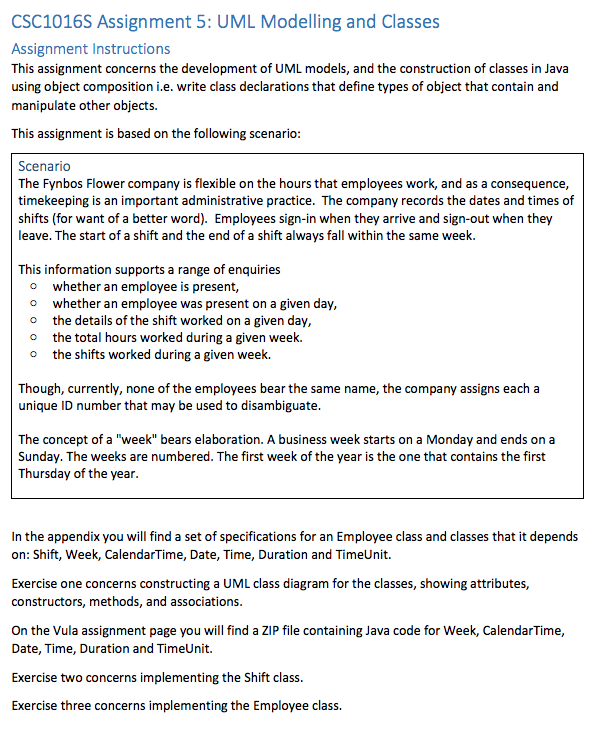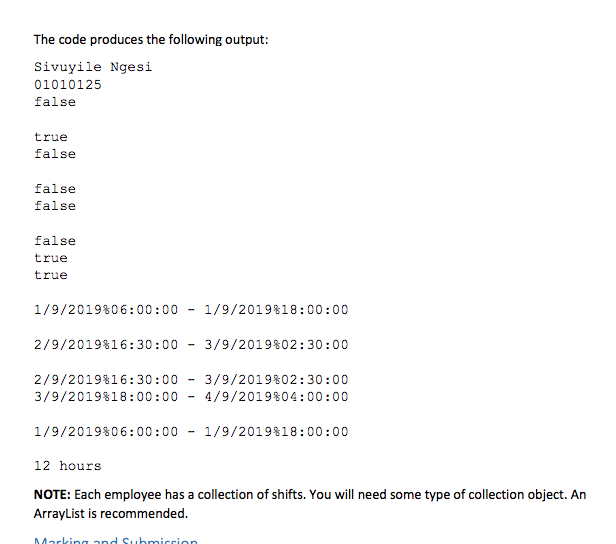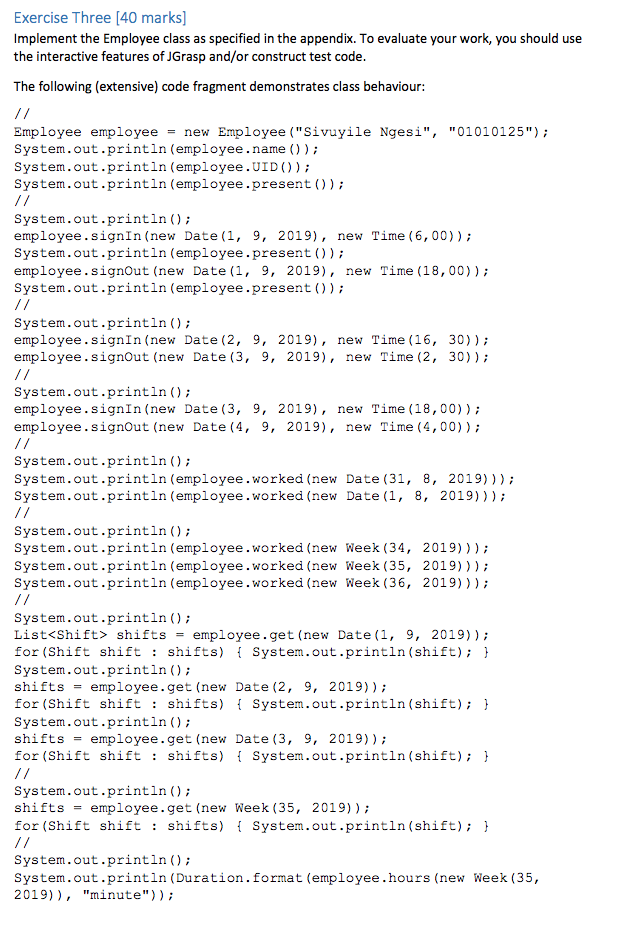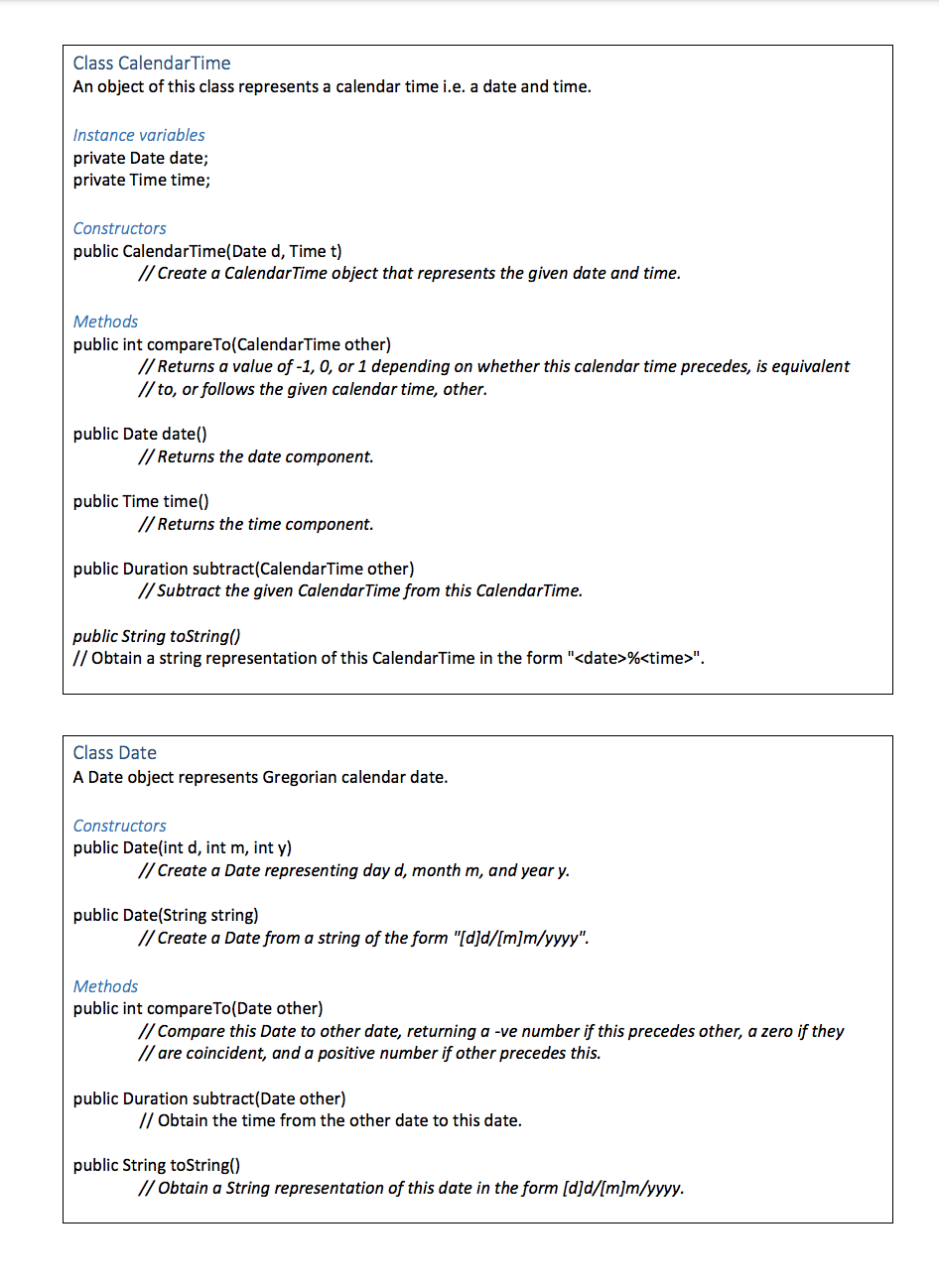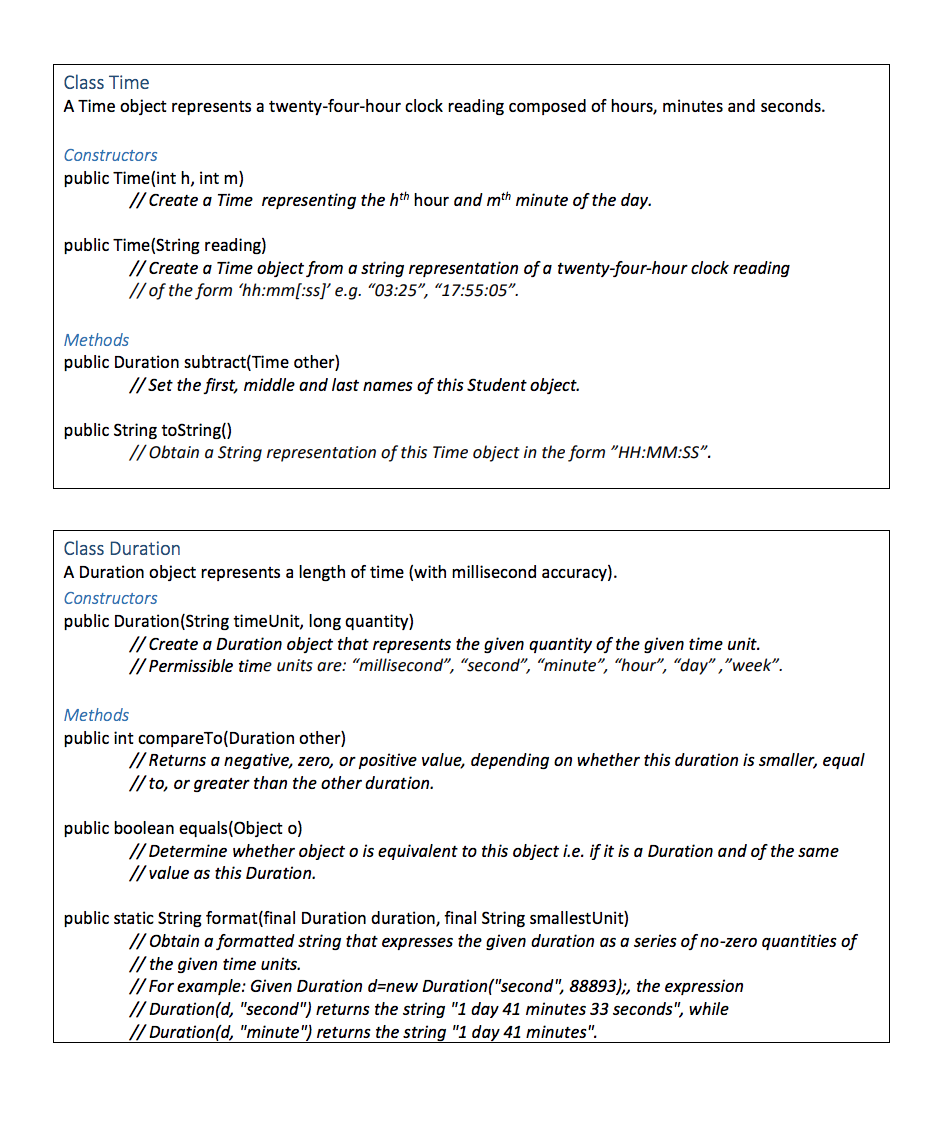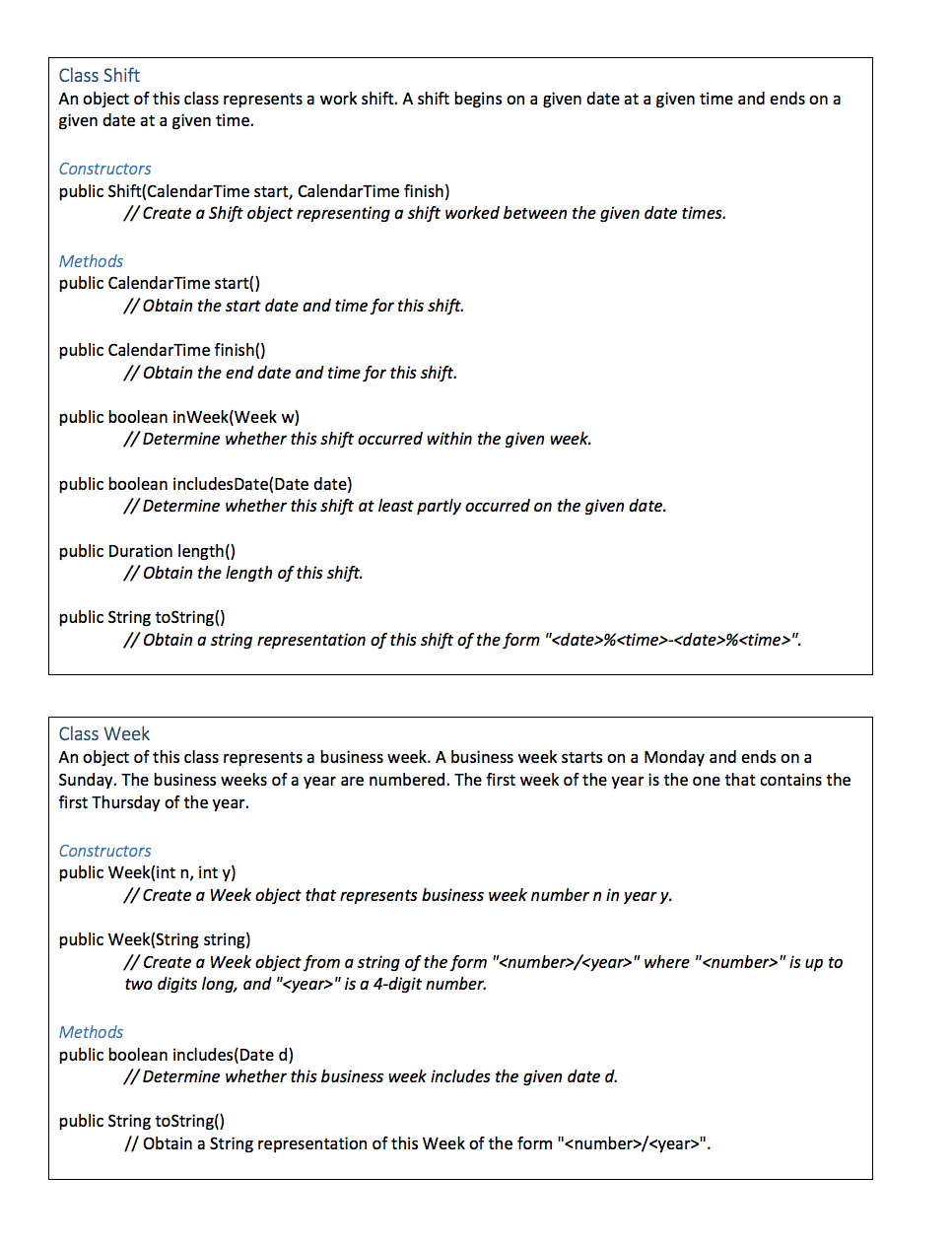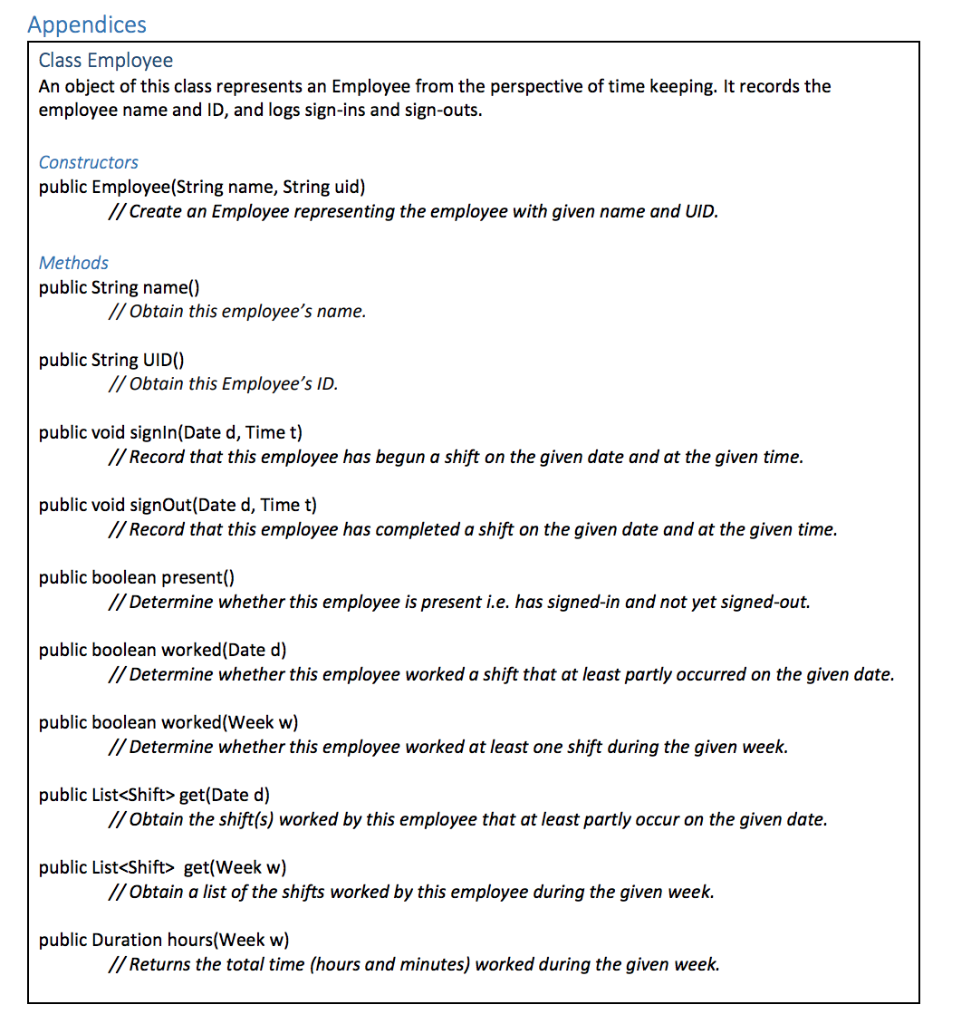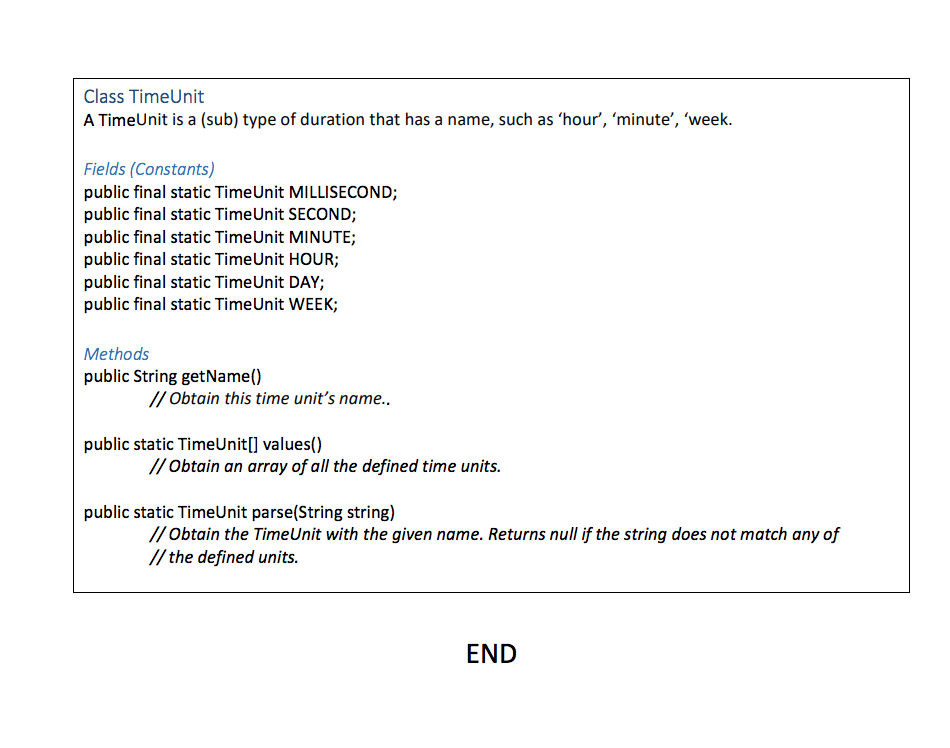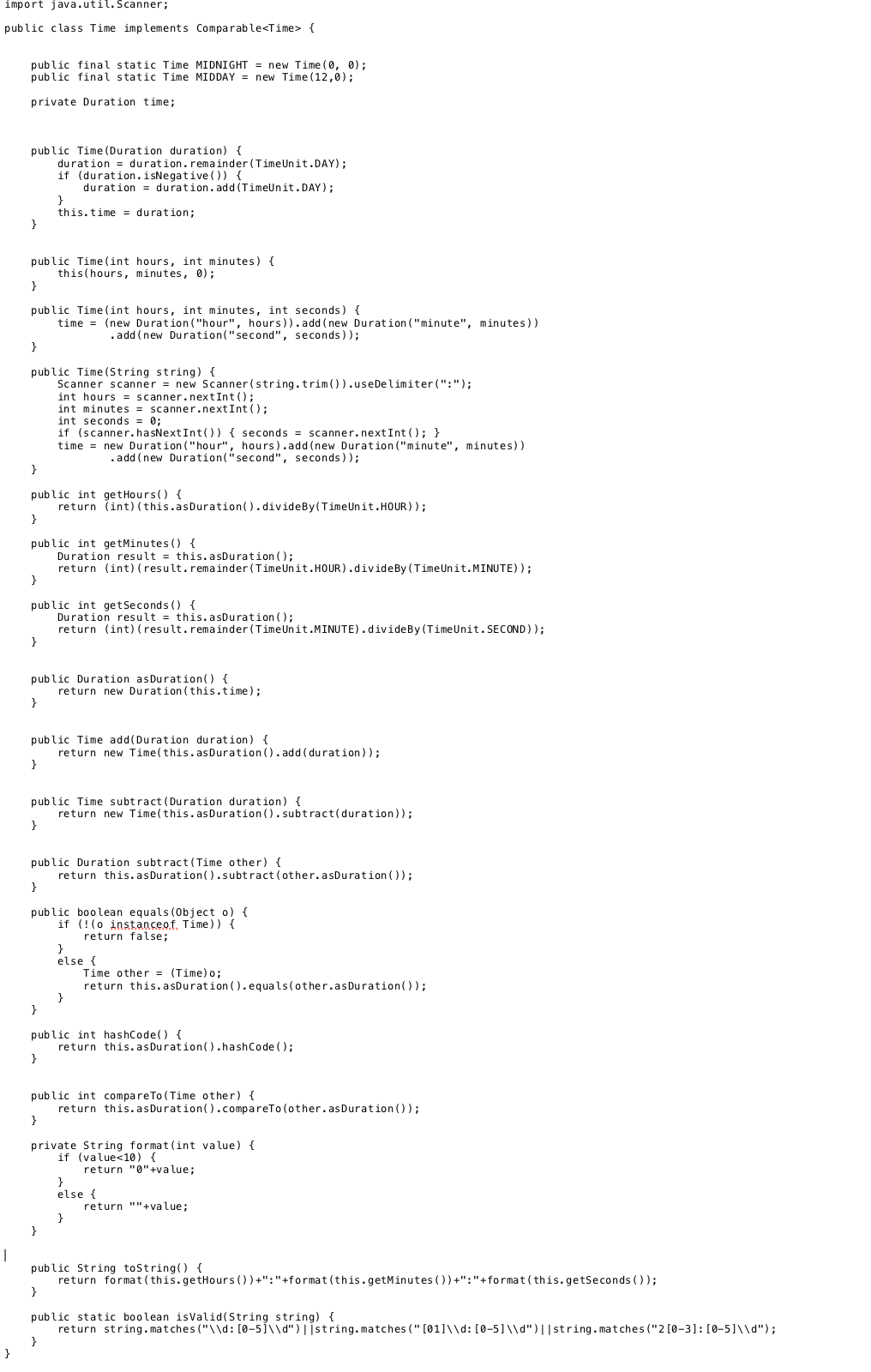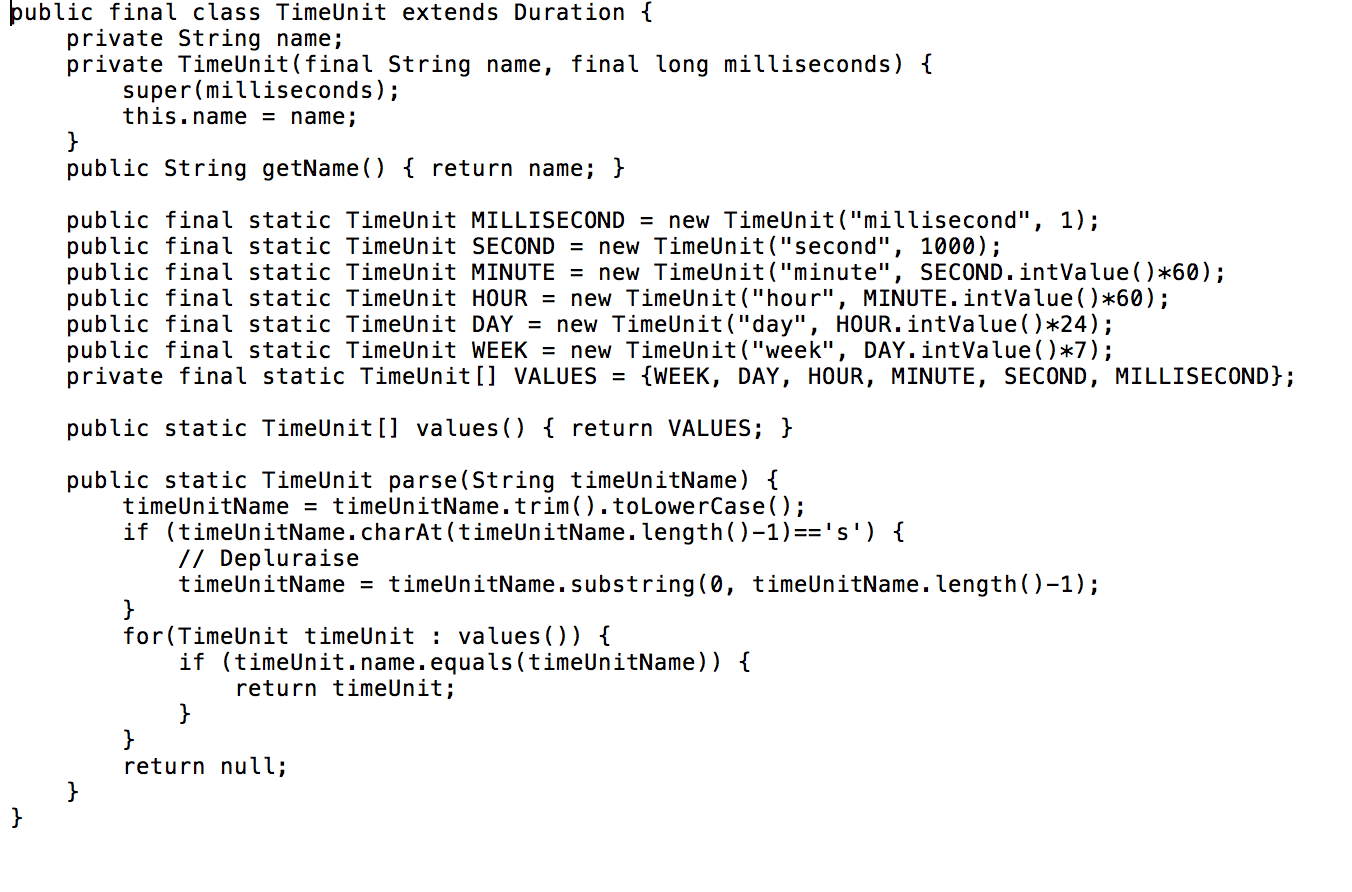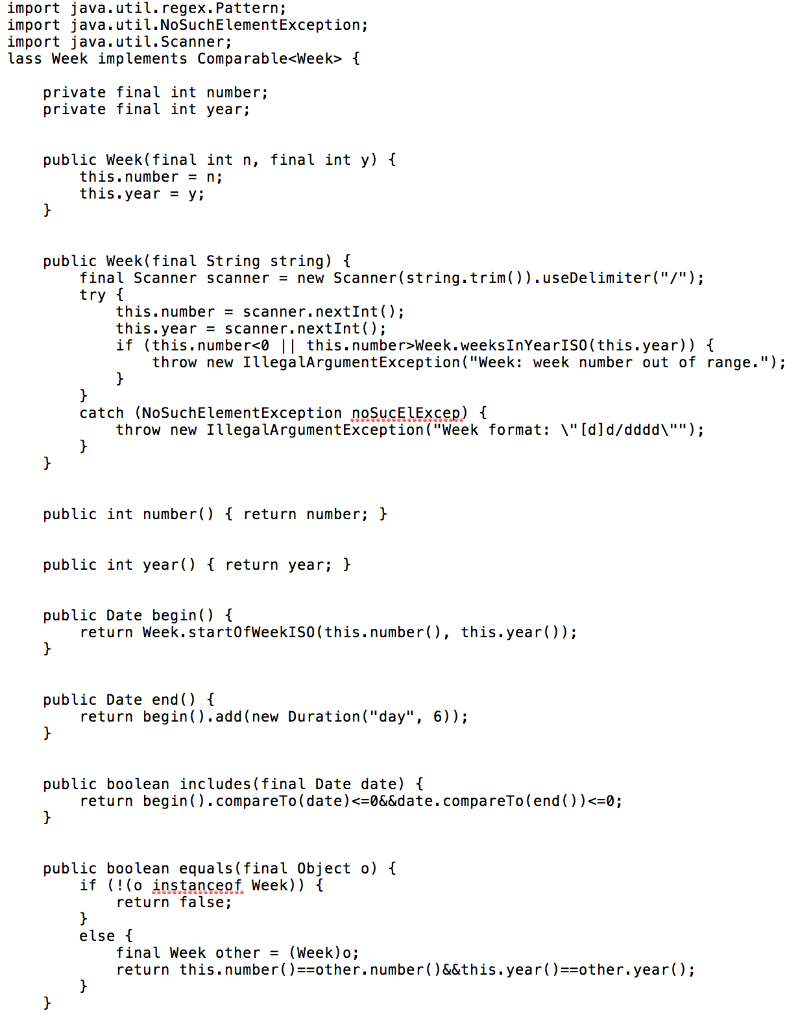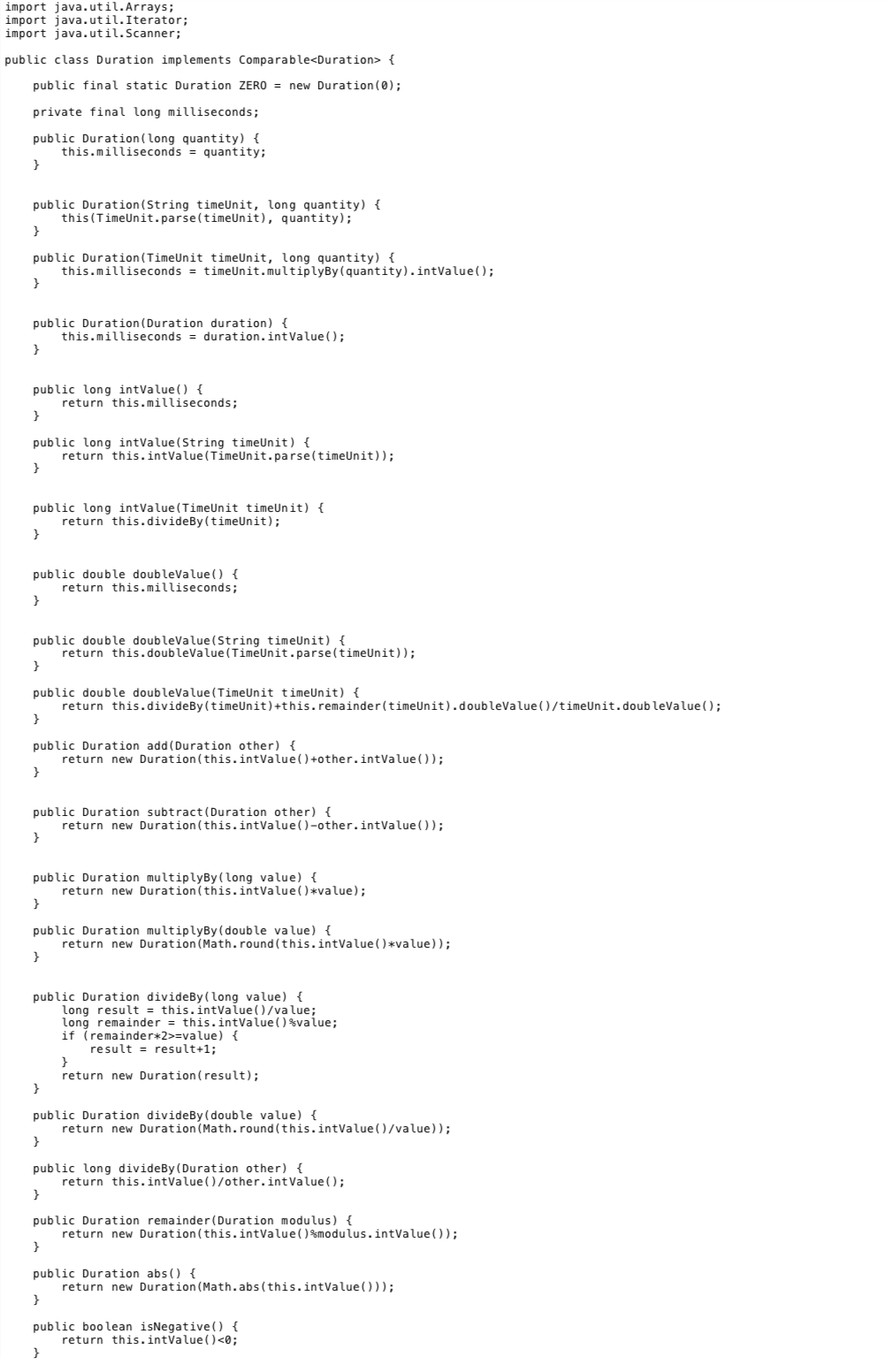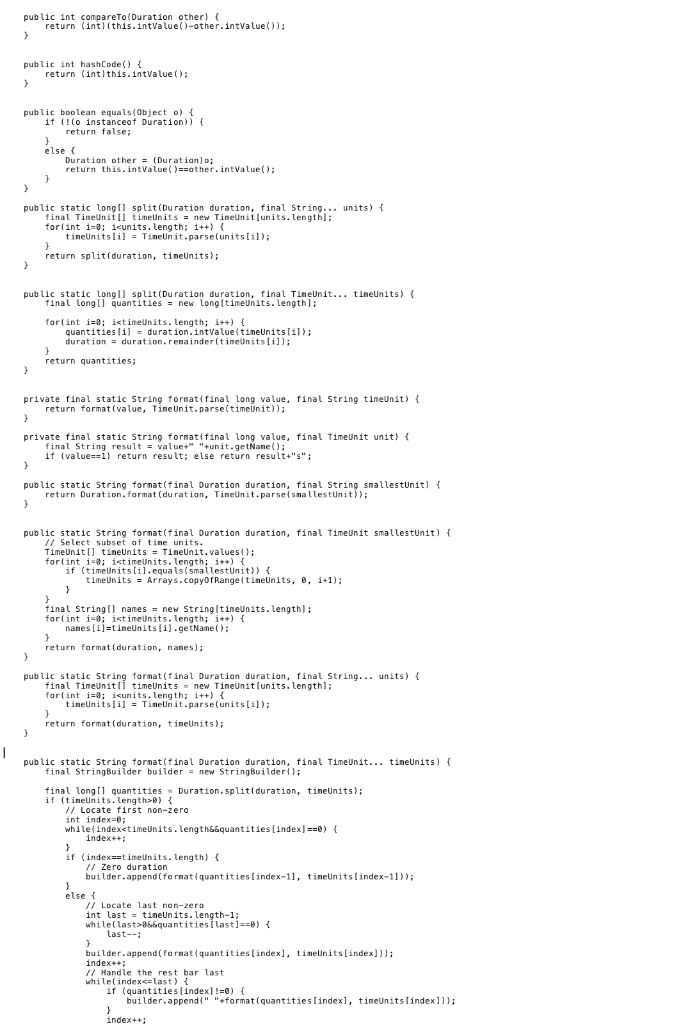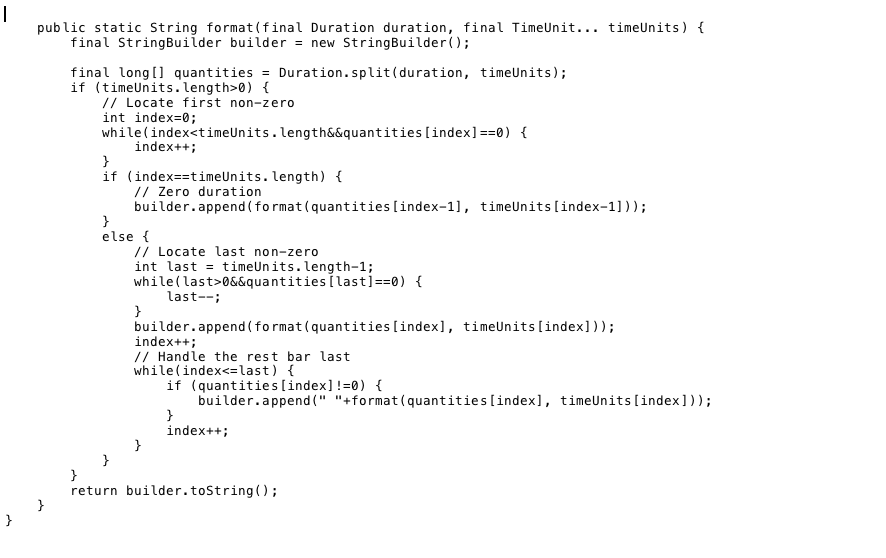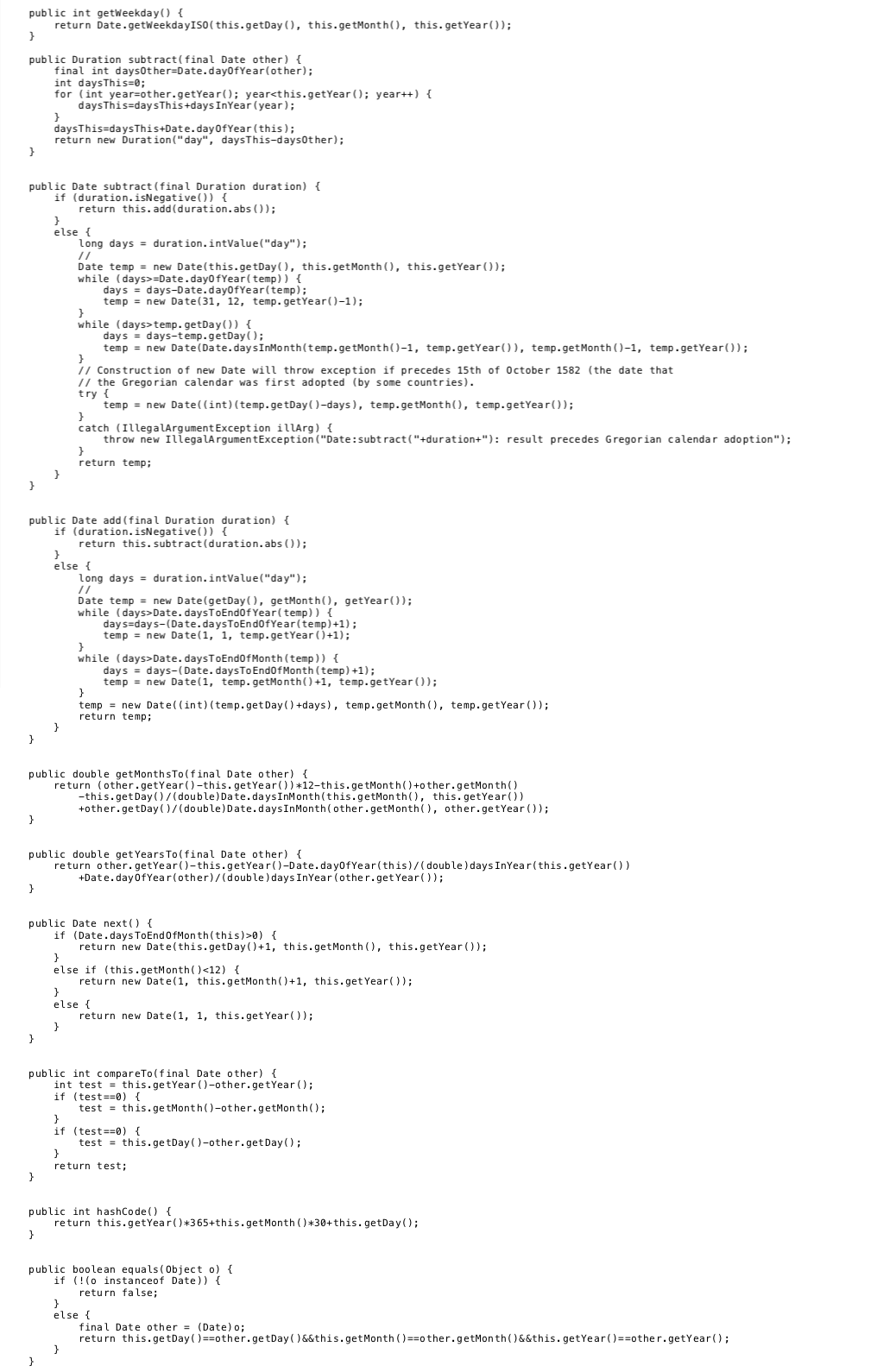Hi there, this is a follow up on my previous question, pleaseagain all the help I can get right now will so be so greatlyappreciated! thank you so so so much

The code produces the following output: Sivuyile Ngesi 01010125 false true false false D false true true 1/9/2019806:00:00 – 1/9/2019818:00:00 2/9/2019816:30:00 – 3/9/2019802:30:00 2/9/2019816:30:00 – 3/9/2019802:30:00 3/9/2019818:00:00 – 4/9/2019804:00:00 1/9/2019806:00:00 – 1/9/2019818:00:00 12 hours NOTE: Each employee has a collection of shifts. You will need some type of collection object. An ArrayList is recommended. Marlinand Submiccion
Exercise Three [40 marks] Implement the Employee class as specified in the appendix. To evaluate your work, you should use the interactive features of JGrasp and/or construct test code. The following (extensive) code fragment demonstrates class behaviour: Employee employee = new Employee (“Sivuyile Ngesi”, “01010125”); System.out.println(employee.name()); System.out.println(employee.UID()); System.out.println(employee.present()); System.out.println(); employee. signIn(new Date(1, 9, 2019), new Time (6,00)); System.out.println (employee. present()); employee.signOut (new Date(1, 9, 2019), new Time (18,00)); System.out.println(employee.present()); System.out.println(); employee.signIn (new Date(2, 9, 2019), new Time (16, 30)); employee. signOut (new Date(3, 9, 2019), new Time (2, 30)); System.out.println(); employee.signIn (new Date(3, 9, 2019), new Time (18,00)); employee.signOut (new Date(4, 9, 2019), new Time (4,00)); System.out.println(); System.out.println (employee.worked (new Date (31, 8, 2019))) ; System.out.println (employee.worked (new Date(1, 8, 2019))); System.out.println(); System.out.println (employee.worked (new Week (34, 2019))); System.out.println (employee.worked (new Week (35, 2019))); System.out.println (employee.worked (new Week (36, 2019))); // System.out.println(); List shifts = employee.get (new Date(1, 9, 2019)); for (Shift shift : shifts) { System.out.println(shift); } System.out.println(); shifts = employee.get (new Date(2, 9, 2019)); for (Shift shift : shifts) { System.out.println(shift); } System.out.println(); shifts = employee.get (new Date(3, 9, 2019)); for (Shift shift : shifts) { System.out.println(shift); } System.out.println(); shifts = employee.get (new Week (35, 2019)); for (Shift shift : shifts) { System.out.println(shift); } System.out.println(); System.out.println (Duration. format (employee hours (new Week (35, 2019)), “minute”));
Class Calendar Time An object of this class represents a calendar time i.e. a date and time. Instance variables private Date date; private Time time; Constructors public CalendarTime(Date d, Time t) // Create a Calendar Time object that represents the given date and time. Methods public int compare To(CalendarTime other) // Returns a value of -1, 0, or 1 depending on whether this calendar time precedes, is equivalent // to, or follows the given calendar time, other. public Date date() // Returns the date component. public Time time() // Returns the time component. public Duration subtract(Calendar Time other) // Subtract the given Calendar Time from this CalendarTime. public String toString() // Obtain a string representation of this Calendar Time in the form “%”. Class Date A Date object represents Gregorian calendar date. Constructors public Date(int d, int m, int y) // Create a Date representing day d, month m, and year y. public Date(String string) // Create a Date from a string of the form “[d]d/[m]m/yyyy”. Methods public int compareTo(Date other) // Compare this Date to other date, returning a -ve number if this precedes other, a zero if they // are coincident, and a positive number if other precedes this. public Duration subtract(Date other) // Obtain the time from the other date to this date. public String toString() // Obtain a String representation of this date in the form [d]d/[m]m/yyyy.
Class Time A Time object represents a twenty-four-hour clock reading composed of hours, minutes and seconds. Constructors public Time(int h, int m) // Create a Time representing the nth hour and mth minute of the day. public Time (String reading) // Create a Time object from a string representation of a twenty-four-hour clock reading // of the form ‘hh:mm[:ss)’e.g. “03:25”, “17:55:05”. Methods public Duration subtract(Time other) // Set the first, middle and last names of this Student object. public String toString() // Obtain a String representation of this Time object in the form “HH:MM:SS”. Class Duration A Duration object represents a length of time (with millisecond accuracy). Constructors public Duration(String timeUnit, long quantity) // Create a Duration object that represents the given quantity of the given time unit. // Permissible time units are: “millisecond”, “second”, “minute”, “hour”, “day”,”week”. Methods public int compareTo(Duration other) // Returns a negative, zero, or positive value, depending on whether this duration is smaller, equal // to, or greater than the other duration. public boolean equals(Object o) // Determine whether object o is equivalent to this object i.e. if it is a Duration and of the same // value as this Duration. public static String format(final Duration duration, final String smallest Unit) // Obtain a formatted string that expresses the given duration as a series of no-zero quantities of // the given time units. // For example: Given Duration d=new Duration(“second”, 88893);, the expression // Duration(d, “second”) returns the string “1 day 41 minutes 33 seconds”, while // Duration(d, “minute”) returns the string “1 day 41 minutes”.
Class Shift An object of this class represents a work shift. A shift begins on a given date at a given time and ends on a given date at a given time. Constructors public Shift(Calendar Time start, CalendarTime finish) // Create a Shift object representing a shift worked between the given date times. Methods public CalendarTime start() // Obtain the start date and time for this shift. public CalendarTime finish() // Obtain the end date and time for this shift. public boolean in Week(Week w) // Determine whether this shift occurred within the given week. public boolean includes Date(Date date) // Determine whether this shift at least partly occurred on the given date. public Duration length() // Obtain the length of this shift. public String toString() // Obtain a string representation of this shift of the form “%-%”. Class Week An object of this class represents a business week. A business week starts on a Monday and ends on a Sunday. The business weeks of a year are numbered. The first week of the year is the one that contains the first Thursday of the year. Constructors public Week(int n, int y) // Create a Week object that represents business week number n in year y. public Week(String string) // Create a Week object from a string of the form “/” where “” is up to two digits long, and “” is a 4-digit number. Methods public boolean includes(Date d) // Determine whether this business week includes the given date d. public String toString() // Obtain a String representation of this Week of the form “/”.
Appendices Class Employee An object of this class represents an Employee from the perspective of time keeping. It records the employee name and ID, and logs sign-ins and sign-outs. Constructors public Employee(String name, String uid) // Create an Employee representing the employee with given name and UID. Methods public String name() // Obtain this employee’s name. public String UIDO) // Obtain this Employee’s ID. public void signIn(Date d, Time t) // Record that this employee has begun a shift on the given date and at the given time. public void signOut(Date d, Time t) // Record that this employee has completed a shift on the given date and at the given time. public boolean present() // Determine whether this employee is present i.e. has signed-in and not yet signed-out. public boolean worked(Date d) // Determine whether this employee worked a shift that at least partly occurred on the given date. public boolean worked(Week w) // Determine whether this employee worked at least one shift during the given week. public Listget(Date d) // Obtain the shift(s) worked by this employee that at least partly occur on the given date. public List get(Week w) // Obtain a list of the shifts worked by this employee during the given week. public Duration hours(Week w) // Returns the total time (hours and minutes) worked during the given week.
Class TimeUnit A TimeUnit is a (sub) type of duration that has a name, such as ‘hour’, ‘minute’, ‘week. Fields (Constants) public final static TimeUnit MILLISECOND; public final static Time Unit SECOND; public final static Time Unit MINUTE; public final static Time Unit HOUR; public final static TimeUnit DAY; public final static TimeUnit WEEK; Methods public String getName() // Obtain this time unit’s name.. public static Time Unit[] values() // Obtain an array of all the defined time units. public static TimeUnit parse(String string) // Obtain the Time Unit with the given name. Returns null if the string does not match any of // the defined units. END
import java.util.Scanner; public class Time implements Comparable { public final static Time MIDNIGHT = new Time (0, 0); public final static Time MIDDAY = new Time (12,0); private Duration time; public Time (Duration duration) { duration = duration. remainder (TimeUnit.DAY); if (duration.isNegative() { duration = duration. add (TimeUnit.DAY); this.time = duration; public Time (int hours, int minutes) { this(hours, minutes, 0); public Time (int hours, int minutes, int seconds) { time = (new Duration (“hour”, hours)).add(new Duration (“minute”, minutes)) .add(new Duration(“second”, seconds)); public Time (String string) { Scanner scanner = new Scanner(string.trim()).useDelimiter(“:”); int hours = scanner.nextInt(); int minutes = scanner.nextInt (); int seconds = 0; if (scanner. hasNextInt() { seconds = scanner.nextInt(); } time = new Duration (“hour”, hours).add(new Duration (“minute”, minutes)) .add(new Duration(“second”, seconds)); public int getHours() { return (int) (this.asDuration().divideBy(TimeUnit.HOUR)); public int getMinutes() { Duration result = this.asDuration(); return (int) (result. remainder (TimeUnit.HOUR).divideBy (TimeUnit. MINUTE)); public int get Seconds() { Duration result = this.asDuration(); return (int) (result. remainder (Time Unit.MINUTE). divideBy (TimeUnit. SECOND)); public Duration as Duration() { return new Duration(this.time); public Time add(Duration duration) { return new Time(this.as Duration().add(duration)); public Time subtract (Duration duration) { return new Time(this.asDuration().subtract(duration)); public Duration subtract (Time other) { return this. as Duration().subtract (other.asDuration()); public boolean equals (Object o) { if (!(o instanceof. Time)) { return false; else { Time other = (Time) o; return this.asDuration().equals(other.asDuration()); public int hashCode() { return this. as Duration().hash Code(); public int compare To (Time other) { return this. as Duration().compareTo (other.as Duration()); private String format (int value) { if (valueDate.daysToEndOf Month (temp)) { days = days-(Date.days To EndofMonth (temp) +1); temp = new Date(1, temp.getMonth() +1, temp.getYear(); temp = new Datel (int) (temp.getDay() +days), temp.getMonth(), temp.getYear()); return temp; public double get Month sto(final Date other) { return (other.getYear()-this.getYear() ) *12-this.getMonth() +other.getMonth() -this.get Day /(double) Date.daysInMonth(this.getMonth(), this.getYear() +other.getDayO/(double) Date.daysInMonth(other.getMonth(), other.getYear(); public double get Years To(final Date other) { return other.getYear()-this.getYear()-Date.dayOfYear(this)/(double) days InYear(this.getYear()) +Date.day Of Year(other)/(double) days In Year (other.getYear(); public Date next() { if (Date.days ToEnd OfMonth(this)>0){ return new Date(this.getDay()+1, this.getMonth(), this.getYear()); else if (this.getMonth()[Image_Link]https://... View full description